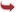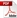International Journal of Scientific and Research Publications

#### IJSRP, Volume 6, Issue 7, July 2016 Edition [ISSN 2250-3153]Models for Intersection and Collision with Earth’s Orbit
Eric Sullivan
Abstract: Data from the article “Equations for Planetary Ellipses” was used in order to create parametric and polar versions of the planetary equations. Using the standard form for both a parametric ellipse and a polar ellipse these equations were written. With the polar equations, collisions and intersections can be determined. In order to see if intersections or collisions were possible, four standard models of orbital interference were created. These models prove that there are potential for objects to collide with Earth. The derivative of each other the orbital interference models was determined in order to know the rate of change at any point. The most useful point would be an intersection or collision point.
[VIEW FULL PAPER][DOWNLOAD]

Reference this Research Paper (copy & paste below code):

Eric Sullivan (2018); Models for Intersection and Collision with Earth’s Orbit; Int J Sci Res Publ 6(7) (ISSN: 2250-3153). http://www.ijsrp.org/research-paper-0716.php?rp=P555665